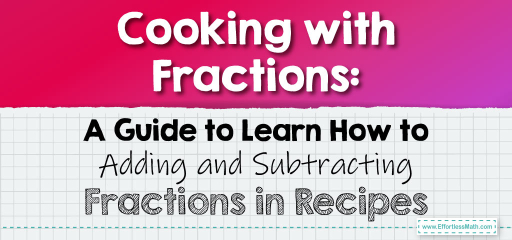# Cooking with Fractions: A Guide to Learn How to Adding and Subtracting Fractions in Recipes

Cooking and baking are as much an art as they are a science. And when it comes to the science part, fractions play a significant role. Whether you're doubling a recipe, making half of it, or combining multiple recipes, understanding how to add and subtract fractions is essential.In this post, we’ll explore the world of culinary math, focusing on adding and subtracting fractions commonly found in recipes.

## Step-by-step Guide to Adding and Subtracting Fractions in Recipes:

1. Recognizing the Need for Fraction Operations:

When adjusting recipe quantities, you’ll often need to add or subtract fractions. For instance, if you’re combining two recipes or halving a recipe, you’ll be working with fractions.

2. Determining the Least Common Denominator (LCD):

Before adding or subtracting, ensure the fractions have the same denominator. If not, find the LCD, which is the smallest number into which all the denominators can divide.

3. Adjusting the Fractions to the LCD:

Modify each fraction so that they all have the LCD as their denominator.

4. Performing the Operation:

Depending on the requirement, either add or subtract the numerators of the fractions to get the final quantity.

### Example 1:

A cookie recipe requires $$\frac{1}{2}$$ cup of sugar. If you’re doubling the recipe, how much sugar will you need?

Solution:

You’ll need to add $$\frac{1}{2}$$ cup + $$\frac{1}{2}$$ cup = $$\frac{2}{2}$$ cup, which equals 1 cup of sugar.

The Absolute Best Book for 5th Grade Students

### Example 2:

You’re making a cake and need to combine two recipes. One recipe requires $$\frac{3}{4}$$ cup of milk, and the other requires $$\frac{1}{2}$$ cup. How much milk is needed in total?

Solution:

The total milk required is $$\frac{3}{4}$$ cup + $$\frac{1}{2}$$ cup. The LCD is 4. Adjusting the fractions:

– $$\frac{3}{4}$$ remains the same.

– $$\frac{1}{2}$$ becomes $$\frac{2}{4}$$.

In total, you’ll need $$\frac{5}{4}$$ cups, which is 1 $$\frac{1}{4}$$ cups of milk.

### Practice Questions:

1. A bread recipe requires $$\frac{1}{3}$$ cup of oil. If you’re making half the recipe, how much oil will you need?

2. You’re combining two pasta recipes. One needs $$\frac{2}{5}$$ cup of cheese, and the other requires $$\frac{3}{10}$$ cup. How much cheese is required in total?

3. A muffin recipe asks for $$\frac{3}{8}$$ cup of blueberries. If you’re tripling the recipe, how many cups of blueberries will you need?

A Perfect Book for Grade 5 Math Word Problems!

1. $$\frac{1}{6}$$ cup

2. $$\frac{4}{5}$$ cup

3. $$\frac{9}{8}$$ cups or 1 $$\frac{1}{8}$$ cups

The Best Math Books for Elementary Students

### What people say about "Cooking with Fractions: A Guide to Learn How to Adding and Subtracting Fractions in Recipes - Effortless Math: We Help Students Learn to LOVE Mathematics"?

No one replied yet.

X
51% OFF

Limited time only!

Save Over 51%

SAVE $15 It was$29.99 now it is \$14.99